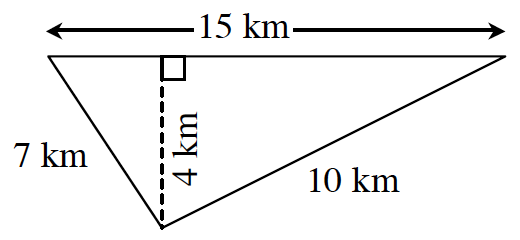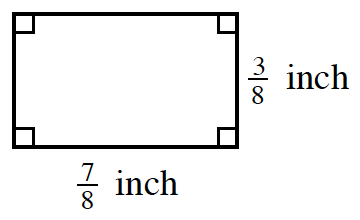### Home > MC2 > Chapter 9 > Lesson 9.2.2 > Problem9-53

9-53.

Find the perimeter and area of each figure.

1.Add all the side lengths together to find the perimeter.

$7+15+10=?$

Use the equation for finding the area of a triangle.

$\text{Area}=\frac{1}{2}\text{ (base)(height)}$

1.Add all the side lengths together to find the perimeter.
A rectangle has two pairs of sides with equal lengths.

$\frac{7}{8}+\frac{3}{8}+\frac{7}{8}+\frac{3}{8}=?$

Use the equation for finding the area of a rectangle.

$\text{Area}=\left(\text{base}\right)\left(\text{height}\right)$

Perimeter: $\frac{20}{8}=2.5$ inches
Area: $\frac{21}{64}$in2